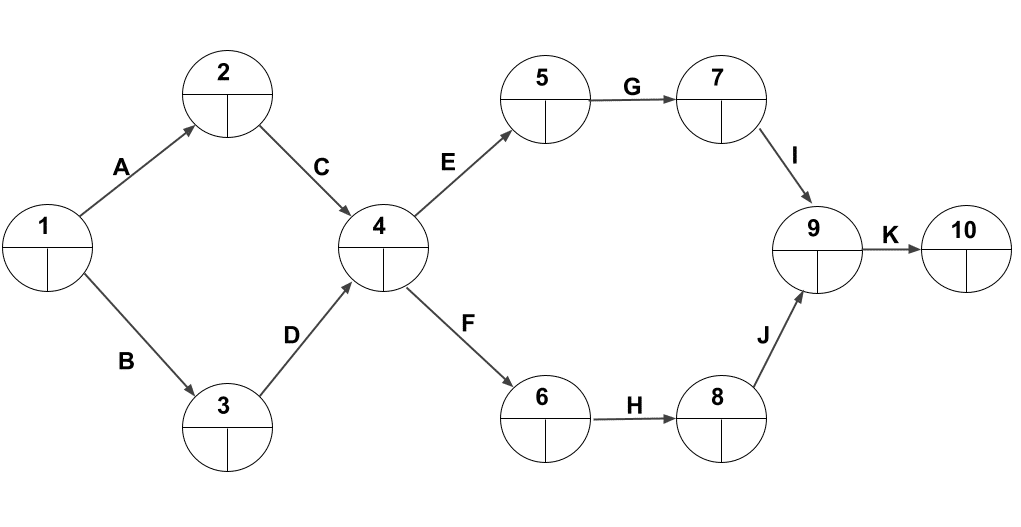# Construct a project network with predecessor relationship | Operation Research | Numerical

## Question: Construct a project network with the following predecessor relationship:

A < C E < G I < K
B < D F < H J < K
C < E, F G < I
D < E, F H < J

## Solution:

• To start with project network, we need to convert this relationships into a simple tabular one.

If you practice well, you can skip the tabular conversion.

## Tabular conversion:

Activity Predecessor activity
A -
B -
C A
D B
E C,D
F C,D
G E
H F
I G
J H
K I,J

We can understand this table as follows:

• For A < C (A less than C); we can say that A must be completed before C
• Same way, for C < E, F and D < E, F; we can say that C and D both must be completed

1. before the start of E and
2. before the start of F

In a similar manner, I have added the whole relationship given in the question in simple activity - predecessor activity format as shown in the table above.Remember:

In case of A < C, the lesser value is the predecessor activity of greater value, i.e. A is the predecessor activity of C.

Also for the activities whose predecessors are not defined are the initial or starting activities of the project.

Now let's generate network diagram from the table (or you can directly do it from the question)Note that, we have left all the cells empty in the event nodes. We will update the question soon with duration and we will solve this using CPM method and find the project completion time along with critical activities and critical path.

To be continued...